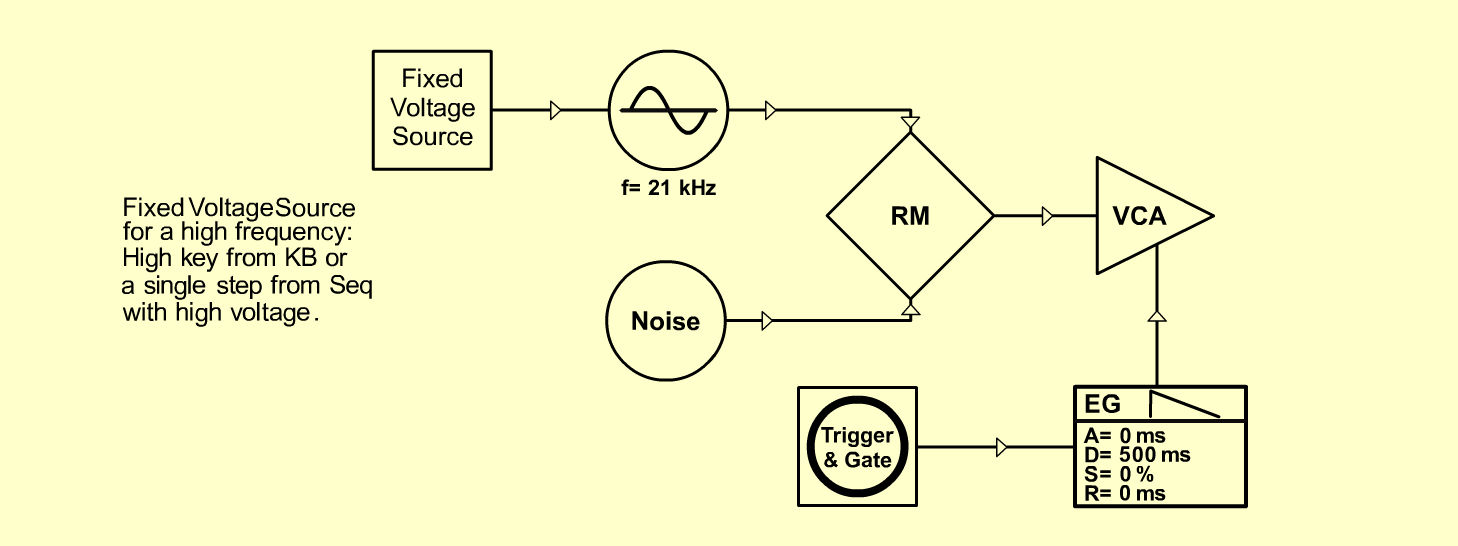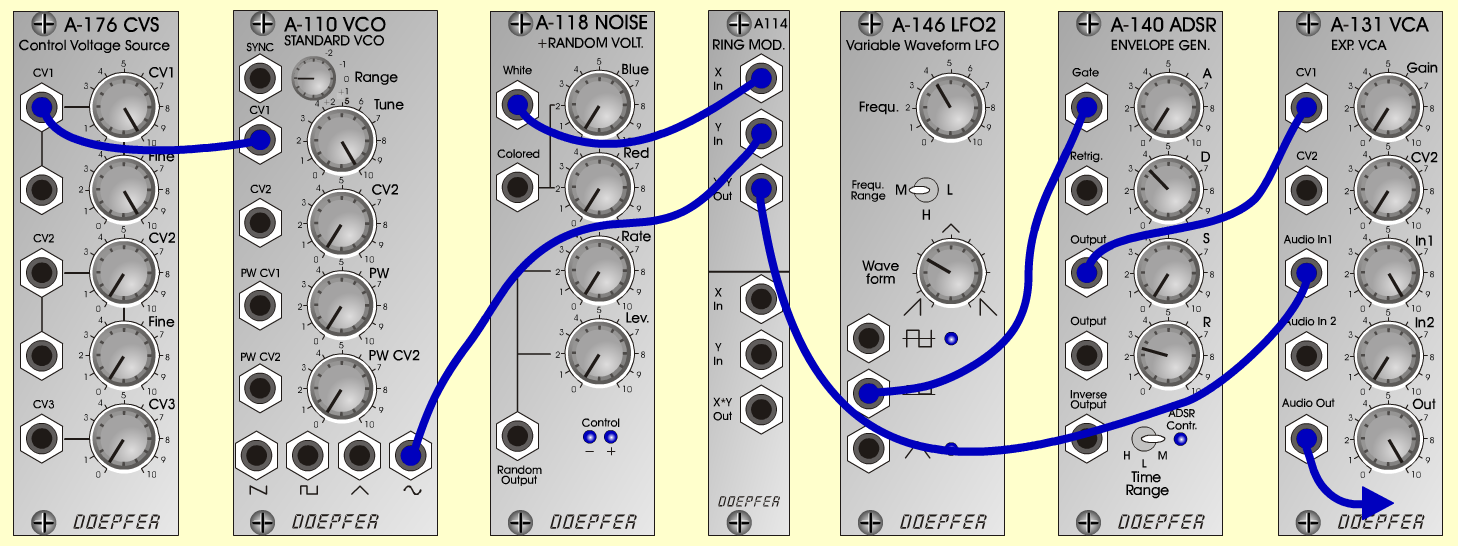TambourineD r u m sD o e p f e r A - 100 Connections: Settings: A-176 (CV 1) <=>   A-110 (CV 1)   A-110 (Sine) <=>   A-114 (X In)   A-118 (White) <=>   A-114 (Y In)   A-114 (Out) <=>   A-131 (Audio In 1)   Trigger and Gate: KB, Seq or   A-146 (Square) <=>   A-140 (Gate)   A-140 (Output) <=>   A-131 (CV 1) A-176 (CV 1 = 10, Fine = 10)   A-110 (Range = +5, Tune = 10)   A-146 (Freq = 4, Wave Form = 3, Range = M)   A-140 (A = 0, D = 3.5, S = 0, R = 2.5, Range = M)   A-131 (Gain = 0, Audio In 1 = 10, Audio Out = 10) Notes: Change pitch with A-176 (CV 1) and A-110    Range).   Try higher values for A-140 (D, R).   Try also A-110 (Triangle and Square) and   A-118 (Colored) Josef MuellerSound sample Tambourine